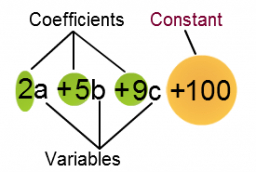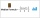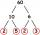# Translate

Translate the following mathematical statement into an algebraic expression or equation.
1. Nine less that the quotient of a number and 3
2. The ratio of 3 and the sum of 4 and an unknown number
3. Eighty is the product of 4 and g
4. The sum of x and 18 is 25
5. p minus 30

Result

f = (Correct answer is: a/3-9)b =  2
g =  20
x =  7
e = (Correct answer is: p-30)### Step-by-step explanation:

$f=a\mathrm{/}3-9$
$e=p-30$Did you find an error or inaccuracy? Feel free to write us. Thank you!Tips to related online calculators
Do you have a linear equation or system of equations and looking for its solution? Or do you have a quadratic equation?

## Related math problems and questions:

• SelectSelect the statement that describes this expression: 5 x (3 + 4) - 4. a) 5 times the sum of 3 and 4, then subtract 4 b) 5 times 3 plus 4 minus 4 c) 5 more than the sum of 3 and 4, and then subtract 4 d) 5 times 3 plus the difference of 3 and 4 What is the
• Expressions with variableThis is algebra. Let n represent an unknown number and write the following expressions: 1. 4 times the sum of 7 and the number x 2. 4 times 7 plus the number x 3. 7 less than the product of 4 and the number x 4. 7 times the quantity 4 more than the number
• ExpressionsLet k represent an unknown number, express the following expressions: 1. The sum of the number n and two 2. The quotient of the numbers n and nine 3. Twice the number n 4. The difference between nine and the number n 5. Nine less than the number n
• Fractions and mixed numerals(a) Convert the following mixed numbers to improper fractions. i. 3 5/8 ii. 7 7/6 (b) Convert the following improper fraction to mixed number. i. 13/4 ii. 78/5 (c) Simplify these fractions to their lowest terms. i. 36/42 ii. 27/45 2. evaluate following ex
• The rawThe raw data presented here are the scores (out of 100 marks) of a market survey regarding the acceptability of new product launched by a company for random sample of 50 respondents: 40 45 41 45 45 30 30 8 48 25 26 9 23 24 26 29 8 40 41 42 39 35 18 25 35
• Algebra problemThis is algebra. Let n represent an unknown number. 1. Eight more than the number n 2. Three times the number n 3. The product of the number n and eight 4. Three less than the number n 5. Three decreased by the number n
• Divisible by 5How many three-digit odd numbers divisible by 5, which are in place ten's number 3?
• Median or middleThe number of hours of television watched per day by a sample of 28 people is given below: 4, 1, 5, 5, 2, 5, 4, 4, 2, 3, 6, 8, 3, 5, 2, 0, 3, 5, 9, 4, 5, 2, 1, 3, 4, 7, 2, 9 What is the median value?
• ComplaintsThe table is given: days complaints 0-4 2 5-9 4 10-14 8 15-19 6 20-24 4 25-29 3 30-34 3 1.1 What percentage of complaints were resolved within 2weeks? 1.2 calculate the mean number of days to resolve these complaints. 1.3 calculate the modal number of day
• Quotient 3If the quotient of 8/13 and 2 is subtracted from the product of 1 3/4 and 8/21, what is the difference?
• ValueDetermine the value of the following exspressions: a) (23-25)·(4-5) b) (97-123):(18+8)
• Three digits number 2Find the number of all three-digit positive integers that can be put together from digits 1,2,3,4 and which are subject to the same time has the following conditions: on one positions is one of the numbers 1,3,4, on the place of hundreds 4 or 2.
• Dataset:Dataset: 35 22 18 54 22 46 28 31 43 22 14 17 25 19 33 14. 1 Group the data into a grouped distribution using 6 classes of equal width. 2. Determine the mean, median, and mode using the raw data. 3. Draw an Ogive curve corresponding to the data and use it
• Z9–I–4 MO 2017Numbers 1, 2, 3, 4, 5, 6, 7, 8 and 9 were prepared for a train journey with three wagons. They wanted to sit out so that three numbers were seated in each carriage and the largest of each of the three was equal to the sum of the remaining two. The conduct
• PoolIf water flows into the pool by two inlets, fill the whole for 19 hours. The first inlet filled pool 5 hour longer than the second. How long pool take to fill with two inlets separately?
• Here isHere is a data set (n=117) that has been sorted. 10.4 12.2 14.3 15.3 17.1 17.8 18 18.6 19.1 19.9 19.9 20.3 20.6 20.7 20.7 21.2 21.3 22 22.1 22.3 22.8 23 23 23.1 23.5 24.1 24.1 24.4 24.5 24.8 24.9 25.4 25.4 25.5 25.7 25.9 26 26.1 26.2 26.7 26.8 27.5 27.6 2
• Find unknown 2Find unknown denominator: 2/3 -5/? = 1/4